### Thread: Wheel of Tolkien

<<                                                                                                                                                   >>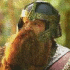Now, Please give me a G if available master!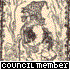Yes,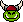we have one B, three Ds, one G, and two Ls for Thorin.

So it now reads:

_ _ _ _ / _ _ _ _ / _ _ _ D / _ _ _ / G _ L D _ _ - _ _ D /

_ _ _ / _ _ _ _ _ / _ _ _ / B _ _ _ / _ L _ _ _ !

Hooray,there are still no incorrect guesses at this time.
some Os?Yes,we have one B, three Ds, one G, and two Ls for Thorin; and eight, count'em eight Os for Thinker.

So it now reads:

_ _ O _ / _ O _ _ / _ _ _ D / _ O _ / G O L D _ _ - _ _ D /

_ _ _ / _ _ O _ _ / _ O _ / B O _ _ / _ L O _ _ !

Hooray,there are still no incorrect guesses at this time.What about a couple of Rs?Yes,we have one B, three Ds, one G, and two Ls for Thorin; and eight, count'em eight Os for Thinker; and four Rs for Thorin.

So it now reads:

_ _ O _ / _ O _ R / _ _ _ D / _ O _ / G O L D _ _ - R _ D /

_ _ _ / _ R O _ _ / _ O _ / B O R _ / _ L O _ _ !

Hooray,there are still no incorrect guesses at this time.

The solution shouldn't take too long now.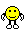N ?A couple of Es to accompany Thinker's N!Yes,we have one B, three Ds, five Es, one G, and two Ls and four Rs for Thorin; and three Ns and eight, count'em eight Os for Thinker.

So it now reads:

_ _ O N / _ O _ R / _ E _ D / _ O _ / G O L D E N - R E D /

_ _ E / _ R O _ N / _ O _ / B O R E / _ L O _ _ !

Hooray,there are still no incorrect guesses at this time.

The solution shouldn't take too long now.RAny F dear Grondy?Yes,we have one B, three Ds, five Es, one F, one G, and two Ls and four Rs for Thorin; and three Ns and eight, count'em eight Os for Thinker.

So it now reads:

_ _ O N / _ O _ R / _ E _ D / _ O _ / G O L D E N - R E D /

_ _ E / _ R O _ N / _ O _ / B O R E / _ L O F _ !

Sorry, :orcssad: Thinker, Thorin correctly guessed the Rs on 05 July.
Then i will ask for some Ss for this summerYes,we have one B, three Ds, five Es, one F, one G, and two Ls and four Rs for Thorin; and three Ns and eight, count'em eight Os for Thinker.

So it still reads:

_ _ O N / _ O _ R / _ E _ D / _ O _ / G O L D E N - R E D /

_ _ E / _ R O _ N / _ O _ / B O R E / _ L O F _ !

Sorry, :orcssad: There is nary an S in my puzzle.
Oh my Bad luck.....mm then Ms?Any W Grondy Sir?Yes,we have one B, three Ds, five Es, one F, one G, and two Ls, four Rs, and two Ws for Thorin; and three Ns and eight, count'em eight Os for Thinker.

So it still reads:

_ _ O N / _ O _ R / _ E _ D / _ O W / G O L D E N - R E D /

_ _ E / _ R O W N / _ O _ / B O R E / _ L O F _ !
]

Sorry, :orcssad: There is nary an M nor an S in my puzzle.
some As?Yes,we have one B, three Ds, five Es, one F, one G, and two Ls, four Rs, and two Ws for Thorin; and two As, three Ns, and eight, count'em eight Os for Thinker.

So it still reads:

_ _ O N / _ O _ R / _ E A D / _ O W / G O L D E N - R E D /

_ _ E / _ R O W N / _ O _ / B O R E / A L O F _ !

Sorry, :orcssad: There is nary an M nor an S in my puzzle.Any H?Yes,we have one B, three Ds, five Es, one F, one G, three Hs, two Ls, four Rs, and two Ws for Thorin; and two As, three Ns, and eight, count'em eight Os for Thinker.

So it now reads:

_ _ O N / _ O _ R / H E A D / H O W / G O L D E N - R E D /

_ H E / _ R O W N / _ O _ / B O R E / A L O F _ !

Sorry, :orcssad: There is nary an M nor an S in my puzzle.
T...?Yes,we have one B, three Ds, five Es, one F, one G, three Hs, two Ls, four Rs, and two Ws for Thorin; and two As, three Ns, eight, count'em eight Os, and two Ts for Thinker.

So it now reads:

_ _ O N / _ O _ R / H E A D / H O W / G O L D E N - R E D /

T H E / _ R O W N / _ O _ / B O R E / A L O F T !

Sorry,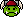There is nary an M nor an S in my puzzle.Yes,we have one B, three Ds, five Es, one F, one G, three Hs, two Ls, four Rs, and two Ws for Thorin; and two As, three Ns, eight, count'em eight Os, and two Ts for Thinker.

So it still reads:

_ _ O N / _ O _ R / H E A D / H O W / G O L D E N - R E D /

T H E / _ R O W N / _ O _ / B O R E / A L O F T !

There are now only four missing letters needed to fill in the remaining seven blanks.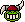Sorry,There is nary an M nor an S in my puzzle.Any C for me?Yes,we have one B, one C, three Ds, five Es, one F, one G, three Hs, two Ls, four Rs, and two Ws for Thorin; and two As, three Ns, eight, count'em eight Os, and two Ts for Thinker.

So it still reads:

_ _ O N / _ O _ R / H E A D / H O W / G O L D E N - R E D /

T H E / C R O W N / _ O _ / B O R E / A L O F T !

There are now only three missing letters needed to fill in the remaining six blanks.Sorry,There is nary an M nor an S in my puzzle.I'd give a hint here, but as there are only three missing letters, l think that would be anti-climatic.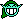I?Anyone want to revive this game by posting a new quiz?No, I isn't one of them

One of the missing letters is U. See if you can now solve it.

It is a good thing it was almost solved, for the solution file expired with my old hard drive.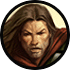I'm sure that the first word is UPON, so I'll put forward the U.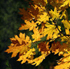Is Y the other letter?
Is this it?

Upon your head, how golden red; The crown you bore aloft.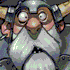You don't know how excited I am now that this thread is up and running again.And, I do believe the above post is correct.
Kaching.

Do I make one now, or is it back to whoever is running this thread?Eruheran has it correct, and may now make for us the next puzzle.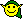The verse is from Bregalad's lament for the rowan-trees ravished by the Orcs. He sang it while the hobbits were waiting at his Ent house during the Entmoot and is found in TTT.
_ _ _ ? _ _ , _ _ _ / _ _ _ _ _ _ _ / _ _ _ _ _ ' _/ _ _ _ / _ _ _ _.
_ _ _ _ _ /_ _ / _ _ _ _ /_ _ _ _ _ _ _ / _ _ _ _ , _ _ _ / _ _ _ _ / _ _ / _ _ _ / _ _ _ _ / _ _ _ _.

This one might look hard, but It is probably quite predictable.

Hint; Similar to a quote from a 900 year old space frog, part of it is.OK... I'll start off with something certain. An E?
E _ _ ? _ _ , _ _ E / _ _ _ _ _ E _ / _ _ E _ _ ' _/ E _ _ / _ E _ E.
_ E _ _ _ /_ _ / _ _ _ _ /_ _ _ _ _ E _ / _ _ _ _ , _ _ E / _ _ _ _ / _ E / _ _ _ / _ _ _ _ / _ _ _ E.

Correct Letters - E
Incorrect Letters -I'll go through the vowels... A?
E _ _ ? _ _ , _ _ E / _ _ _ _ _ E _ / _ _ E _ _ ' _/ E _ _ / _ E _ E.
_ E A _ _ /_ _ / _ _ _ _ /A _ _ _ _ E _ / _ A _ _ , _ _ E / _ _ _ _ / _ E / A _ _ / _ _ _ _ / _ A _ E.

Correct Letters - E, A
Incorrect Letters -Might there possibly be any Ns?
E N _ ? N _ , _ _ E / _ _ _ _ N E _ / _ _ E _ N ' _/ E N _ / _ E _ E.
_ E A _ _ /_ _ / _ _ _ _ /A N _ _ _ E _ / _ A _ _ , _ N E / _ _ _ _ / _ E / A _ _ / _ _ _ _ / _ A _ E.

Correct Letters - E, A, N
Incorrect Letters -Are there any Ss ?
E N _ ? N _ , _ _ E / _ _ _ _ N E _ / _ _ E S N ' _/ E N _ / _ E _ E.
_ E A _ _ /_ S / _ _ S _ /A N _ _ _ E _ / _ A _ _ , _ N E / _ _ _ _ / _ E / A _ _ / _ _ S _ / _ A _ E.

Correct Letters - E, A, N, S
Incorrect Letters -Can I have an I?
E N _ ? N _ , _ _ E / _ _ _ _ N E _ / _ _ E S N ' _/ E N _ / _ E _ E.
_ E A _ _ / Is / _ _ S _ /A N _ _ _ E _ / _ A _ _ , _ N E / _ _ _ _ / _ E / A _ _ / _ _ S _ / _ A _ E.

Correct Letters - E, A, N, S, I
Incorrect Letters -Please, there must be a few Ds in your puzzle.

End? N _ , _ _ E / _ _ _ _ N E _ / D _ E S N ' _/ End / _ E _ E.
D E A _ _ / Is / _ _ S _ /A N _ _ _ E _ / _ A _ _ , _ N E / _ _ _ _ / _ E / A _ _ / _ _ S _ / _ A _ E.

Correct Letters - E, A, N, S, I, D
Incorrect Letters -Got it!
A beautiful quote that you gave, Eruheran, and one of the lines from the films which were moving yet did not come from Tolkien himself.

Gandalf: End? No, the journey doesn't end here. Death is just another path, one that we all must take.(continued) The grey rain-curtain of this world rolls back, and all turns to silver glass... And then you see it.
Pippin: What, Gandalf? See what?
Gandalf: White shores... and beyond, a far green country under a swift sunrise.
Pippin: Well, that isn't so bad.
Gandalf: No... no, it isn't.
<<                                                                                                                                                   >>